# Find voltages across resistors using voltage division

Marcin H

7a from picture

V=IR
Voltage division

## The Attempt at a Solution

I am not sure how to apply voltage division here. It is a new concept for me and I'm not really sure how to apply it here. voltage division can only be used in series, but these resistors are not in series. I also am not sure how to deal with the 2 voltage sources. Is there a way to add them to make this simpler? What do I do with the 10V source exactly? I could find a current, but wouldn't it be only through the one loop on the left? I=V/R=.08A.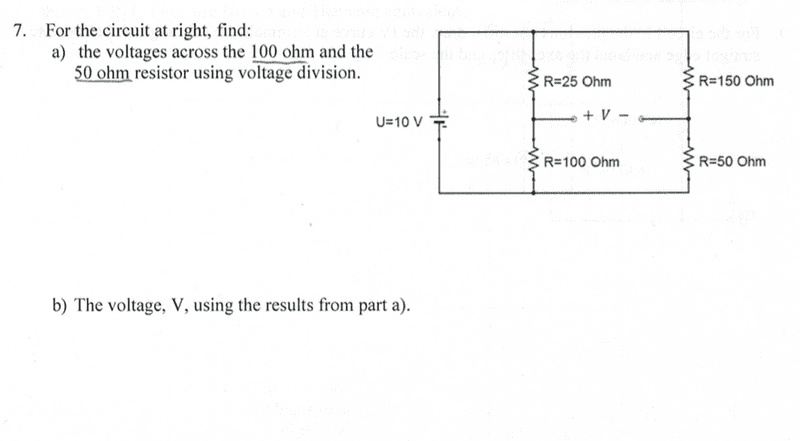Homework Helper
Gold Member
Assume the negative terminal of the battery at 0V. Can you find the current through the two branches?

Homework Helper
Gold Member
I also am not sure how to deal with the 2 voltage sources.
There is only one source, 10V. V is the voltage between the two points shown in figure, which they're asking. Assume it's a voltmeter.

•Marcin H
Marcin H
Assume the negative terminal of the battery at 0V. Can find the current through the two branches?
What do you mean by that? "Assume the negative terminal of the battery at 0V"? Should I ignore the spot on the right where I am supposed to find the voltage for now? And just try finding the equivalent resistance and current?

Homework Helper
Gold Member
What do you mean by that? "Assume the negative terminal of the battery at 0V"? Should I ignore the spot on the right where I am supposed to find the voltage for now? And just try finding the equivalent resistance and current?
Let's keep ground aside for a minute. You've found the current in one branch correctly. What will be the current through the other branch?

Homework Helper
Gold Member
You can simply use Ohms law to find the current through each resistor.

You can then use Ohms law to find the voltage across each resistor.

(BTW. I would stick to the original concept of voltage between two points and not assign any absolute potentials.)

•Marcin H
Marcin H
Let's keep ground aside for a minute. You've found the current in one branch correctly. What will be the current through the other branch?
Ok, so I just ignore the V on the right for now? I thought that was like a wire and it would mess with the circuit. The current for the first loops is .08A and for the second loop it is .05 A.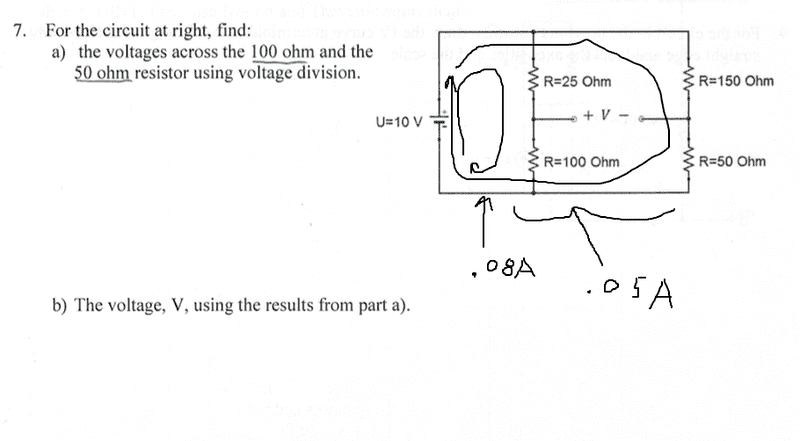Homework Helper
Gold Member
Ok, so I just ignore the V on the right for now? I thought that was like a wire and it would mess with the circuit. The current for the first loops is .08A and for the second loop it is .05 A.
View attachment 95076
Correct. Now as Merlin said earlier, find the voltage drops across 50Ω and 100Ω using Ohm's law.

Homework Helper
Gold Member
Yes. Now you know the current through each resistor, you can find the voltage across them.

Marcin H
Yes. Now you know the current through each resistor, you can find the voltage across them.
Ok. I got 8V for the 100ohm and 2.5V for the 50ohm using ohms law. So now how can I find the voltage V across those 2 wires?
Edit: Voltage in parallel is the same. So what would happen if you connect those to wires and try to find the voltage across them?

Homework Helper
Gold Member
Ok. I got 8V for the 100ohm and 2.5V for the 50ohm using ohms law. So now how can I find the voltage V across those 2 wires?
Yes. If you assume 0V at the negative terminal of the battery, what will be the potentials of the two points( upper ends of the resistors)?

Marcin H
Yes. If you assume 0V at the negative terminal of the battery, what will be the potentials of the two points( upper ends of the resistors)?
I am not sure. What do you mean by upper ends of the resistors?

Homework Helper
Gold Member
I am not sure. What do you mean by upper ends of the resistors?
Label the points as A and B between which the voltage is asked(upper ends of 50ohm and 100 ohm). Label the negative terminal as G. You have VA-VG and VB-VG. Can you find VA-VB from this?

Homework Helper
Gold Member
V is not the voltage at a point. It is the voltage difference between the points labelled + and -

Homework Helper
Gold Member
Edit: Voltage in parallel is the same. So what would happen if you connect those to wires and try to find the voltage across them?
If you connect those points by a wire, it will be a short and voltage between + and - will be 0. It will change everything.

Marcin H
If you connect those points by a wire, it will be a short and voltage between + and - will be 0. It will change everything.
Ok so you just treat this like you are using a voltmeter and measuring the voltage across those 2 wires right?

Label the points as A and B between which the voltage is asked(upper ends of 50ohm and 100 ohm). Label the negative terminal as G. You have VA-VGand VB-VG. Can you find VA-VB from this?
I am not really following what you are doing here. Do I find the voltage at point by using equivalent resistances and then the same at point B and then subtract? So at point A the voltage is V=IReq= (.08A)(125ohms). and then do the same at point B?

Homework Helper
Gold Member
Ok so you just treat this like you are using a voltmeter and measuring the voltage across those 2 wires right?
Right.
Do I find the voltage at point by using equivalent resistances and then the same at point B and then subtract? So at point A the voltage is V=IReq= (.08A)(125ohms). and then do the same at point B?
No. If you labelled correctly, + is A, - is B and negative terminal of battery(where lower ends of 100Ω and 50Ω meet)is G.
You got voltage across 100Ω as 8V. Isn't it VA-VG?

Marcin H
Right.

No. If you labelled correctly, + is A, - is B and negative terminal of battery(where lower ends of 100Ω and 50Ω meet)is G.
You got voltage across 100Ω as 8V. Isn't it VA-VG?
I'm still not following where you are getting these equations or how they are related. Is there a different approach? Or can you explain you approach more?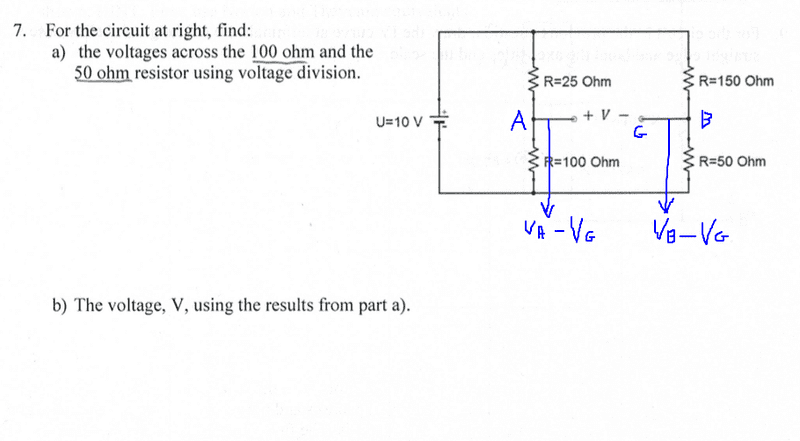Homework Helper
Gold Member
I'm still not following where you are getting these equations or how they are related. Is there a different approach? Or can you explain you approach more? View attachment 95079
Ok. I got your confusion. G is the negative terminal of the battery i.e. the 10V source.

Homework Helper
Gold Member
Is there a different approach? Or can you explain you approach more?
It is the only approach. I am sorry I am unable to draw anything but it is not really difficult. 100 ohm and 50 ohm have a common terminal, don't they? It is connected to the -ve tetminal of 10V source, isn't it? That common terminal is G. Now, voltage "across" 100Ω means VA-VG i.e. potential difference between A and G, right? Same goes with VB-VG. It is the voltage across 50 ohm resistor.

Marcin H
It is the only approach. I am sorry I am unable to draw anything but it is not really difficult. 100 ohm and 50 ohm have a common terminal, don't they? It is connected to the -ve tetminal of 10V source, isn't it? That common terminal is G. Now, voltage "across" 100Ω means VA-VG i.e. potential difference between A and G, right? Same goes with VB-VG. It is the voltage across 50 ohm resistor.
I still don't understand where this is all coming from. Can you show me the equation/work that you do to get the voltage across those 2 points. Maybe I will understand the math better. I can't follow how you are labelling the circuit.

Homework Helper
Gold Member
Can you say what this voltmeter would read?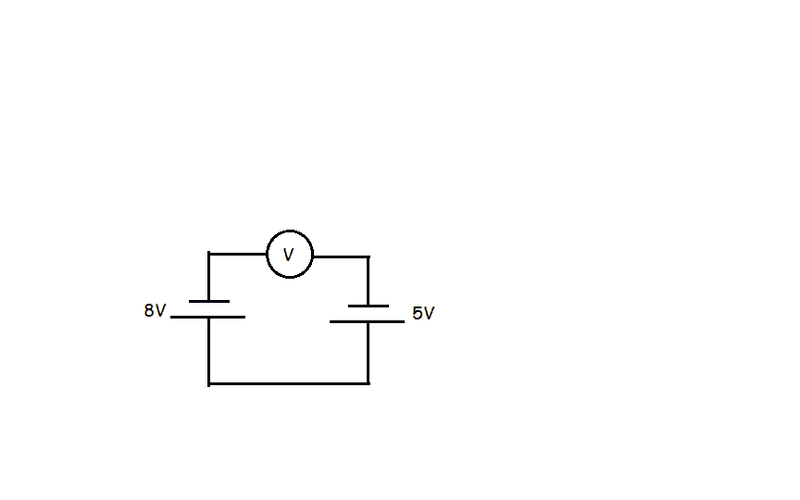Homework Helper
Gold Member
Ok. I got 8V for the 100ohm and 2.5V for the 50ohm using ohms law.
This means voltage "across" 100Ω is 8V and that "across" 50Ω is 2.5V. In the diagram, + is A and - is B(you have labelled them correctly). Do you see the negative terminal of the 10V source is connected to bottom ends of 100Ω and 50Ω using a wire? That wire is point G. Are you aware of the fact that all the points on a wire are equipotential? That means, the common ends of 100 ohm and 50 ohm are at same potential, i.e. VG.

Marcin H
Can you say what this voltmeter would read?View attachment 95081
3V
This means voltage "across" 100Ω is 8V and that "across" 50Ω is 2.5V. In the diagram, + is A and - is B(you have labelled them correctly). Do you see the negative terminal of the 10V source is connected to bottom ends of 100Ω and 50Ω using a wire? That wire is point G. Are you aware of the fact that all the points on a wire are equipotential? That means, the common ends of 100 ohm and 50 ohm are at same potential, i.e. VG.
Ok. So like this? What are Va and Vb then?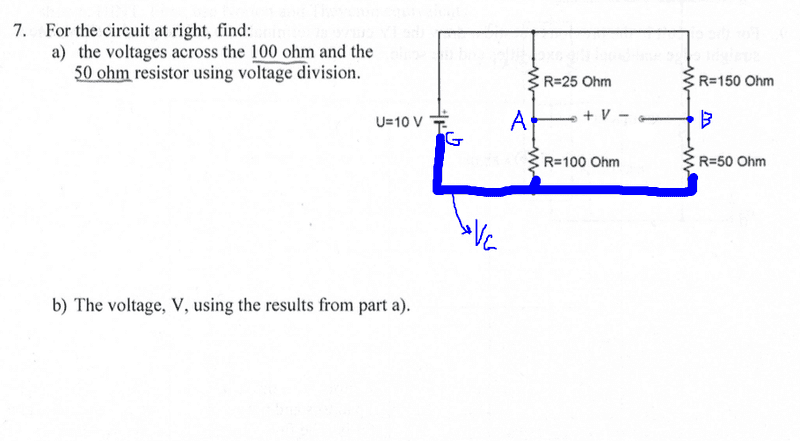Homework Helper
Gold Member
Ok. So like this? What are Va and Vb then?
Right.

Homework Helper
Gold Member
You have the value of Va-VG and VB-VG. What will be VA-VB then?

Marcin H
You have the value of Va-VG and VB-VG. What will be VA-VB then?
Va-Vg=8V and Vb-Vg=2.5V? Using those equations, solve for Va-Vb and get 5.5V?

Homework Helper
Gold Member
Va-Vg=8V and Vb-Vg=2.5V? Using those equations, solve for Va-Vb and get 5.5V?
Exactly! If you assume VG=0, you'll get absolute values of Va and Vb as 8V and 2.5V. The difference is 5.5V.

•Marcin H
Homework Helper
Gold Member
See? Wasn't that difficult!Marcin H
See? Wasn't that difficult!It makes a little more sense now, but not 100%. The math makes sense, but the other stuff is still a bit confusing. Oh well. Thanks for the help!

Homework Helper
Gold Member
The math makes sense, but the other stuff is still a bit confusing.
What do you find confusing? A circuit works with potential "differences". In your circuit, the source is of 10V. Now, all the voltages present in the circuit are due to that 10V source, right? There is always a reference point in a circuit, called 'ground'. All the voltages are measured w.r.t ground. The choice of ground is ours i.e. we can assume it at any point. Here, for the sake of convenience, ground is assumed at the -ve terminal of the 10V source. Now, when you say voltage across 100 ohm is 8V, it is the potential difference between ground and point A, right? And 2.5V is the potential difference between ground and B. Since ground is common to both the resistors, you can say, A is 8V above the ground potential and B is 2.5V above the ground potential. It's like saying building A is 8m tall and building B is 2.5m tall( building height is measured from common ground, isn't it?). So, difference in height between buildings A and B would be 5.5m, right? Similarly, voltage between A and B is 5.5V.

Last edited:
•Marcin H and Merlin3189
Marcin H
Since ground is common to both the resistors, you can say, A is 8V above the ground potential and B is 2.5V above the ground potential.
That's the part that kinda bugged me. I always think of voltage/potential across something or between 2 points. I wasn't sure why you can just say point A is at this potential and point B is at that potential. But that makes sense now. the potential in that portion of the wire is 8V so that means point A is at 8V right? Same for point B?

Homework Helper
Gold Member
the potential in that portion of the wire is 8V so that means point A is at 8V right? Same for point B?
Yes, assuming VG=0V.

•Marcin H